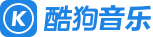#【麦兜是只猪】 正在直播

MAMAMOO - Where R U (你在哪里)
﻿[id:\$00000000] [ar:MAMAMOO] [ti:Where R U] [by:] [hash:444eb7314ff9f7b9d497cc806d617cb2] [al:] [sign:] [qq:] [total:188055] [offset:0] [00:00.01]MAMAMOO - Where R U [00:00.74]作词：코스믹 사운드(RBW)、코스믹 걸、문별 [00:00.88]作曲：코스믹 사운드(RBW)、코스믹 걸 [00:02.10]编曲：코스믹 사운드(RBW)、코스믹 걸 [00:00.27]Guitar：박기태 [00:00.90]Keyboard：코스믹 사운드 [00:01.31]Chorus：코스믹 걸 [00:00.17]Mixed：마스터키 @ 821 Sound [00:03.13]Where are you where are you where are you [00:08.10]Where are you where are you where are you [00:14.58]Where are you [00:20.54]이 넓은 세상 어딘가에 [00:23.64]나만을 바라봐 줄 [00:26.17]누구보다도 예뻐해 줄 [00:28.93]유치하대도 [00:30.50]아니라 해도 그럴 일 없다 해도 [00:33.07]믿고 싶은 걸 그런 널 기다려 [00:36.80]I'm waiting for you [00:39.58]어디야 넌 넌 넌 넌 뭐 하냐고 [00:44.29]묻고 싶어 난 난 난 [00:46.92]I wanna know wanna know [00:50.93]어느 날 갑자기 내게 와 줘 빨리 [00:55.83]그래 넌 어딨니 [00:59.32]Baby [01:00.64]Where are you where are you where are you [01:03.07]Where are you where are you where are you [01:06.21]와요 uh uh uh 내게로 와요 [01:10.66]기다리고 있어 난 여기서 [01:12.99]내가 찾는 넌 어딨어 [01:14.81]Where are you oh where are you [01:19.59]I'm here [01:20.49]지금도 늦지 않았단 말이야 [01:23.06]단둘이 밥 먹고 영화 볼래 uh [01:24.93]생각만 해도 입꼬리는 올라가 [01:27.11]잠이 안 온다면 전화할게 [01:28.73]어디야 pick up the phone [01:30.55]아니라 해도 그럴 일 없다 해도 [01:32.93]믿고 싶어 또 [01:33.97]속고 또 속아도 [01:36.75]이젠 만나고 싶어 [01:39.33]어디야 넌 넌 넌 넌 뭐 하냐고 [01:44.33]묻고 싶어 난 난 난 [01:47.15]I wanna know wanna know oh oh [01:50.85]어느 날 갑자기 내게 와 줘 빨리 [01:55.86]대체 넌 어딨니 [01:59.10]Baby [02:00.60]Where are you where are you where are you [02:03.03]Where are you where are you where are you [02:06.12]와요 uh uh uh 내게로 와요 [02:10.52]기다리고 있어 난 여기서 [02:12.89]내가 찾는 넌 어딨어 [02:14.69]Where are you oh where are you [02:18.08]Oh where are you [02:20.22]이름 모를 너와 [02:23.91]내가 같은 맘 이길 [02:29.13]어느 곳에 있든지 [02:30.96]한눈에 나를 알아볼 수 있게 [02:36.38]별처럼 반짝이듯이 [02:39.04]널 기다리고 있을게 [02:42.21]So where are you where are you where are you [02:45.53]Where are you where are you where are you [02:48.67]와요 uh uh uh 내게로 와요 [02:53.01]기다리고 있어 난 여기서 [02:55.48]내가 찾는 넌 어딨어 [02:57.14]Where are you oh where are you where are you
/

Mac版酷狗音乐已更新